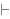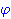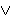Mathbox for BJ < Previous   Next > Nearby theorems Mirrors  >  Home  >  ILE Home  >  Th. List  >   Mathboxes  >  nndc Unicode version

Theorem nndc 10722
 Description: Double negation of decidability of a formula. Intuitionistic logic refutes undecidability (but, of course, does not prove decidability) of any formula. (Contributed by BJ, 9-Oct-2019.)
Assertion
Ref Expression
nndcDECIDProof of Theorem nndc
StepHypRef Expression
1 nnexmid 10721 . 22 df-dc 777 . . 3DECID32notbii 627 . 2DECID41, 3mtbir 629 1DECIDColors of variables: wff set class Syntax hints:wn 3wo 662  DECID wdc 776 This theorem was proved from axioms:  ax-1 5  ax-2 6  ax-mp 7  ax-ia1 104  ax-ia2 105  ax-ia3 106  ax-in1 577  ax-in2 578  ax-io 663 This theorem depends on definitions:  df-bi 115  df-dc 777 This theorem is referenced by:  dcdc  10723
 Copyright terms: Public domain W3C validator